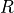# sfepy.discrete.common.dof_info module¶

Classes holding information on global DOFs and mapping of all DOFs - equations (active DOFs).

Helper functions for the equation mapping.

class sfepy.discrete.common.dof_info.DofInfo(name)[source]

Global DOF information, i.e. ordering of DOFs of the state (unknown) variables in the global state vector.

append_raw(name, n_dof)[source]

Append raw DOFs.

Parameters
namestr

The name of variable the DOFs correspond to.

n_dofint

The number of DOFs.

append_variable(var, active=False)[source]

Append DOFs of the given variable.

Parameters
varVariable instance

The variable to append.

activebool, optional

When True, only active (non-constrained) DOFs are considered.

get_info(var_name)[source]

Return information on DOFs of the given variable.

Parameters
var_namestr

The name of the variable.

get_n_dof_total()[source]

Return the total number of DOFs of all state variables.

get_subset_info(var_names)[source]

Return global DOF information for selected variables only. Silently ignores non-existing variable names.

Parameters
var_nameslist

The names of the selected variables.

update(name, n_dof)[source]

Set the number of DOFs of the given variable.

Parameters
namestr

The name of variable the DOFs correspond to.

n_dofint

The number of DOFs.

class sfepy.discrete.common.dof_info.EquationMap(name, dof_names, var_di)[source]

Map all DOFs to equations for active DOFs.

get_operator()[source]

Get the matrix operatorcorresponding to the equation mapping, such that the restricted matrixcan be obtained from the full matrixby. All the matrices are w.r.t. a single variables that uses this mapping.

Returns
mtxcoo_matrix

The matrix.

map_equations(bcs, field, ts, functions, problem=None, warn=False)[source]

Create the mapping of active DOFs from/to all DOFs.

Parameters
bcsConditions instance

The Dirichlet or periodic boundary conditions (single condition instances). The dof names in the conditions must already be canonized.

fieldField instance

The field of the variable holding the DOFs.

tsTimeStepper instance

The time stepper.

functionsFunctions instance

The registered functions.

problemProblem instance, optional

The problem that can be passed to user functions as a context.

warnbool, optional

If True, warn about BC on non-existent nodes.

Returns
active_bcsset

The set of boundary conditions active in the current time.

Notes

• Periodic bc: master and slave DOFs must belong to the same field (variables can differ, though).

sfepy.discrete.common.dof_info.expand_nodes_to_dofs(nods, n_dof_per_node)[source]

Expand DOF node indices into DOFs given a constant number of DOFs per node.

sfepy.discrete.common.dof_info.expand_nodes_to_equations(nods, dof_names, all_dof_names)[source]

Expand vector of node indices to equations (DOF indices) based on the DOF-per-node count.

DOF names must be already canonized.

Returns
eqarray

The equations/DOF indices in the node-by-node order.

sfepy.discrete.common.dof_info.group_chains(chain_list)[source]

Group EPBC chains.

sfepy.discrete.common.dof_info.is_active_bc(bc, ts=None, functions=None)[source]

Check whether the given boundary condition is active in the current time.

Returns
activebool

True if the condition bc is active.

sfepy.discrete.common.dof_info.resolve_chains(master_slave, chains)[source]

Resolve EPBC chains - e.g. in corner nodes.